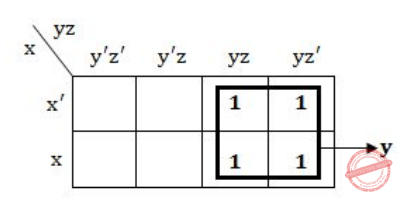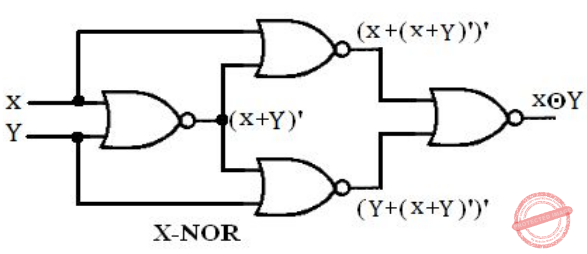## UGC NET CS 2005 june-paper-2

 Question 1
Which of the following is not true ?
 A Power of deterministic automata is equivalent to power of non-deterministic automata. B Power of deterministic pushdown automata is equivalent to power of non-deterministic pushdown automata. C Power of deterministic turing machine is equivalent to power of non-deterministic turing machine. D All the above
Theory-of-Computation       Finite-Automata
Question 1 Explanation:
→ NPDA(Non deterministic Pushdown Automata) and DPDA(Deterministic Pushdown Automata) are not equivalent in power.
→ NPDA is more powerful than DPDA which means for every language for which a dpda exist, there exist an NPDA but there are some languages that are accepted by NPDA but are not accepted by DPDA.
→ In case of DFA and NFA they are equivalent in power. So for every language accepted by DFA there exists an NFA and vice versa.
Hence,we can’t convert NPDA to DPDA always and we can convert NFA to an equivalent DFA always.
→ In case of DTM and NTM they are equivalent in power.
 Question 2
Identify the language which is not context-free.
 A L = { wwR ǀ wε{0,1}* } B L = { a​ n​ b​ n​ ǀ n≥0 } C L = { ww ǀ wε{0,1}* } D L = { a​ n​ b​ m​ c​ m​ d​ n​ ǀ n,m ≥0 }
Theory-of-Computation       Context-Free-Language
Question 2 Explanation:
Option-A​ is a CFL because for this we can have a non deterministic pushdown automata which will accept the string by exploring all the possibilities on each input character.
Option-B​ is correct because we can have a deterministic pushdown automata which will push all the "a" as they come as input and when "b" comes as input for every "b" one "a" will be popped. So in this way we can design a pushdown automata which will accept a​ n​ b​ n​ . Hence a​ n​ b​ n​ is a CFL.
Option-C​ is not a CFL because we can't design a pushdown which can accept CFL. Suppose a string "abcabc". Now let's say pushdown automata recognize w=abc after symbol "c" now when "a" come as input after "c" the pushdown automata have to pop "a" i.e. initial input symbol "a" but top of the stack contains "c" as top element. So in this way we can't have any pushdown automata which can accept "ww" language. So it is not a CFL.
Option-D​ is a CFL because we can design a pushdown automata for a​ n​ b​ m​ c​ m​ d​ n​ . The push down automata will push "a" and "b" as the come as input symbol. When "c" came as input then for each "c" one "b" is popped from top of the stack. And when "d" will come as input symbol the top of stack will contain only a's so for every "d" one "a" is popped . In this way we can design a pushdown automata for a​ n​ b​ m​ c​ m​ d​ n​ .
 Question 3
The transitive closure of a relation R on set A whose relation matrixis
 ABCDEngineering-Mathematics       Set-Theory
Question 3 Explanation:
The transitive closure of R is obtained by repeatedly adding (a,c) to R for each (a,b) ∈ R and (b,c) ∈ R.
 Question 4
Consider the relation on the set of non-negative integers defined by ​ x ​ ≡ ​ y if and only if :
 A x mod 3=3 mod y B 3 mod x≡3 mod y C x mod 3=y mod 3 D None of the above
Engineering-Mathematics       Set-Theory
Question 4 Explanation:
A relation R is an equivalence relation if and only if it is reflexive, symmetric, and transitive.
1. The relation is reflexive: x mod 3 = x mod 3
2. The relation is symmetric: if x mod 3 = y mod 3, then y mod 3 = x mod 3
3. The relation is transitive: if x mod 3 = y mod 3, and y mod 3 = z mod 3, then x mod 3 = z mod 3
 Question 5
Minimum number of individual shoes to be picked up from a dark room ( containing 10 pair of shoes) if we have to get at least one proper pair :
 A 2 B 20 C 11 D None of these
Engineering-Mathematics       Combinatorics
Question 5 Explanation:
→ There are 10 pair of shoes available in the dark room which means 20 individual shoes are available in the room.
→ If you pick shoes from one to ten individual shoes, there may be chance of getting same individual shoes.
→ There is no guarantee that getting one proper pair from 10 individual shoes.
→ If You pick 11 shoes, then there may chance of 10 individual shoes of same type and one individual shoe of another type. So we will get at least one proper pair shoe from 11 individual shoes.
 Question 6
(101011)​ 2​ =(53)​ b​ , then ‘b’ is equal to :
 A 4 B 8 C 10 D 16
Digital-Logic-Design       Number-Systems
Question 6 Explanation:
We are dividing binary number into 3 then
101 011
5 3
(101011)​ 2 = (53)​ 8
Suppose, we are dividing binary digits into 4 then
0010 1011
2 B
So, it is not hexadecimal number.
 Question 7
The logic expression x’yz’+x’yz+xyz’+xyz reduces to :
 A x’z B xyz C y D yz
Digital-Logic-Design       Boolean-Expression
Question 7 Explanation:
We can use two methods to solve this problem.
Method-1 using K-Maps:Method-2 using boolean simplification:
x’yz’+x’yz+xyz’+xyz
= x’y(z’+z)+xy(z’+z)
= x’y + xy
= y(x’+x)
= y
 Question 8
Which of the following logic has the maximum fan out ?
 A RTL B ECL C NMOS D CMOS
Digital-Logic-Design       Logic-Families
Question 8 Explanation:
Emitter Coupled Logic (ECL)
The storage time is eliminated as the transistors are used in difference amplifier mode and are never driven into saturation.
1. Fastest among all logic families
2. Lowest propagation delay.
Complementary metal oxide semiconductor(CMOS)
The power dissipation is usually 10nW per gate depending upon the power supply voltage, output load etc.
1. Lowest power dissipation
2. Excellent noise immunity
3. High packing density
4. Wide range of supply voltage
5. Highest fan out among all logic families
Negative channel metal oxide semiconductor (NMOS)
It is a type of semiconductor that charges negatively. So that transistors are turned ON/OFF by the movement of electrons. In contrast, Positive channel MOS-PMOS works by moving electron vacancies. NMOS is faster than PMOS.
Resistor Transistor Logic (RTL)
Sometimes also transistor–resistor logic (TRL) is a class of digital circuits built using resistors as the input network and bipolar junction transistors (BJTs) as switching devices. RTL is the earliest class of transistorized digital logic circuit used; other classes include diode–transistor logic (DTL) and transistor–transistor logic (TTL).
 Question 9
Which of the following binary number is the same as its 2’s complement :
 A 1010 B 0101 C 1000 D 1001
Digital-Logic-Design       Number-Systems
Question 9 Explanation:
Option-A: 1010 convert into 2’s complement is
1010
1’s complement: 0101
2’s complement: 1
--------
0110
--------- Option-B: 0101 convert into 2’s complement is
0101
1’s complement: 1010
2’s complement: 1
--------
1011
---------
Option-C: 1000 convert into 2’s complement is
1000
1’s complement: 0111
2’s complement: 1
--------
1000
---------
Option-D: 1001 convert into 2’s complement is
1001
1’s complement: 0110
2’s complement: 1
--------
0111
---------
 Question 10
Identify the logic function performed by the circuit shownA Exclusive-OR B AND C Exclusive-NOR D NOR
Digital-Logic-Design       Logic-Gates
Question 10 Explanation:Question 11
After 3 calls of the c function bug( ) below, the values of i and j will be :
int j=1;
bug( )
{
static int i=0; int j=0;
i++;
j++;
return (i);
}
 A i = 0, j = 0 B i = 3, j = 3 C i = 3, j = 0 D i = 3, j = 1
Programming       Storage-Classes
Question 11 Explanation:
Step-1: Initially i=0 but given as static. The scope is lifetime.
Step-2: Call-1: i=1 and j=1
Call-2: i=2 and j=1 because ‘i’ is static ‘j’ is again become 0 when it starts new call.
Call-3: i=3 and j=1 because ‘i’ is static ‘j’ is again become 0 when it starts new call.
Note: A static ‘int’ variable remains in memory while the program is running.
 Question 12
Find the output of the following “C” code :
main ( )
{
int x=20, y=35;
x= y++ + x++;
y= ++y + ++x;
printf (“%d, %d\n”, x,y);
}
 A 55, 93 B 53, 97 C 56, 95 D 57, 94 E 56, 93
Programming       Operator
Question 12 Explanation:
Step-1: Here, we are using post increment for both x and y. In post increment the value assign first and update after assigning.
x= 35 + 20
y= 37 + 56 (In this statement, ‘y’ will become 36 before performing pre increment)
Step-2: Final x=56 and y=93
Note: They given wrong options. We are added correct option.
Excluded for evaluation.
There are 12 questions to complete.

Register Now